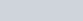# Limit of a function | Concept Booster | IIT-JEE, Class-11 notes

• 5
•
•
•
• 3
•
•
•
8
Shares

 Some equations can go out of the Mobile screen, Use desktop view for convenience.

In mathematics the concept of the limit of a function is one of the fundamental concepts as it provides the base for defining the derivative of a function hence it’s can be seen as an important concept for learning calculus.

# Limit of a function

## Basic Ideas

The concept of limit of a function analyses the behavior of a function around a particular point say x=a.

Let’s Analyse a function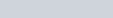now we’re analysing the function around x=2 and graph is given.

We can see if we take values close to 2 whether it’s less than 2 or more the value of the function is around 4. So we can say that if x approaches 2 the value of function approaches 4.

## Defining Limit

In the above example, we can see that as x approaches 2 f(x) approaches 4. One can note out that we can reach x=2 either from the left-hand side or right-hand side of the real line, hence we arrive to define the left-hand limit and right-hand limit of a function.

### Left Hand Limit of a function

Left hand limit(L.H.L.) at x=a of a function is the value(if it exists) towards which function approaches as x approaches from left hand side.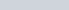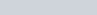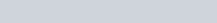### Right Hand Limit

Right hand limit(R.H.L) of a function at x=a is the value towards which function approaches as x approaches a from right hand side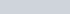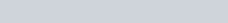### Existence of Limit of a function

Limit of a function f(x) at x = a, is said to be exist if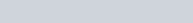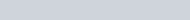It should be noted that-
• “L” is called limiting value of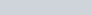• if one of the L.H.L / R.H.L or both doesn’t exist then the limit of function won’t exist.

Limit of a function at a point may exist or may not exist irrespective of whether the function is defined or not defined at that point.

# Fundamental theorems

Following are some fundamental theorems on limits one can see proof for satisfaction.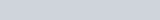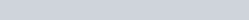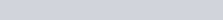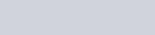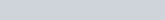It should be ensured that function “f” should be continuous at limiting value of function “g”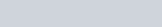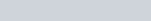# Indeterminate Forms

While evaluating the limit of a function we may come across several of the following listed Indeterminate Forms, these are basically expressions in which we have an ambiguity to directly answer which value will the function attains.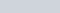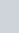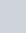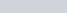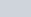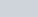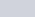It’s noteworthy thatmeans tending to, the terms are tending(approaching) and one should not consider these terms to be a fixed value these are approaching.

# Evaluation of Limit of functions

To evaluate the limiting value of a function at a particular point, one of the abstract ways is to plot the curve of the function and observe the behavior of the function, using the Definition of limit one can find the limit.
But sometimes it’s very tedious to sketch the curve of some functions hence, limits of functions are generally found by an approach as described here.
• if the given form of expression whose limit is to be found is determinate form, then simply analyze the behavior of the function, Calculate LHL and RHL separately hence limit can be found.
• if the expression is an Indeterminate form then we have to simplify it to a determinate form.
For such simplifications, following results and expressions can be used.

## Some useful results for evaluation of Limit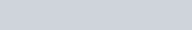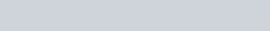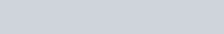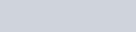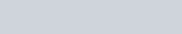## Standard Limits

Following are some standard results stated without proof which are used mostly.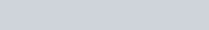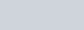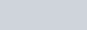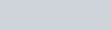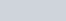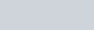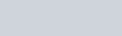## Series Expansions

Sometimes these expansions can be used to evaluate limit. For ex- some Trigonometric expressions can be replaced by algebraic expansions hence it’s useful to know . these results are obtained by maclaurin series (Taylor series)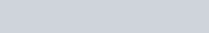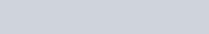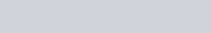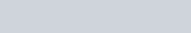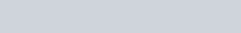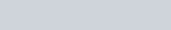## L- Hopital Rule

Suppose in finding limit we got indeterminate from of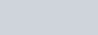or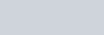in both of these indeterminate forms the limit of function is found by L-Hopital Rule as follows :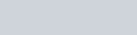## Sandwich Theorem

Also known as Squeeze theorem, Pinching theorem, police theorem, many times squeeze lemma all these refer to Sandwich theorem. It states that-

If we have  f, g and h three real functions such that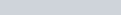holds true for all real values of  x in common domain.

Now for a real number “a” if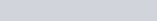Then